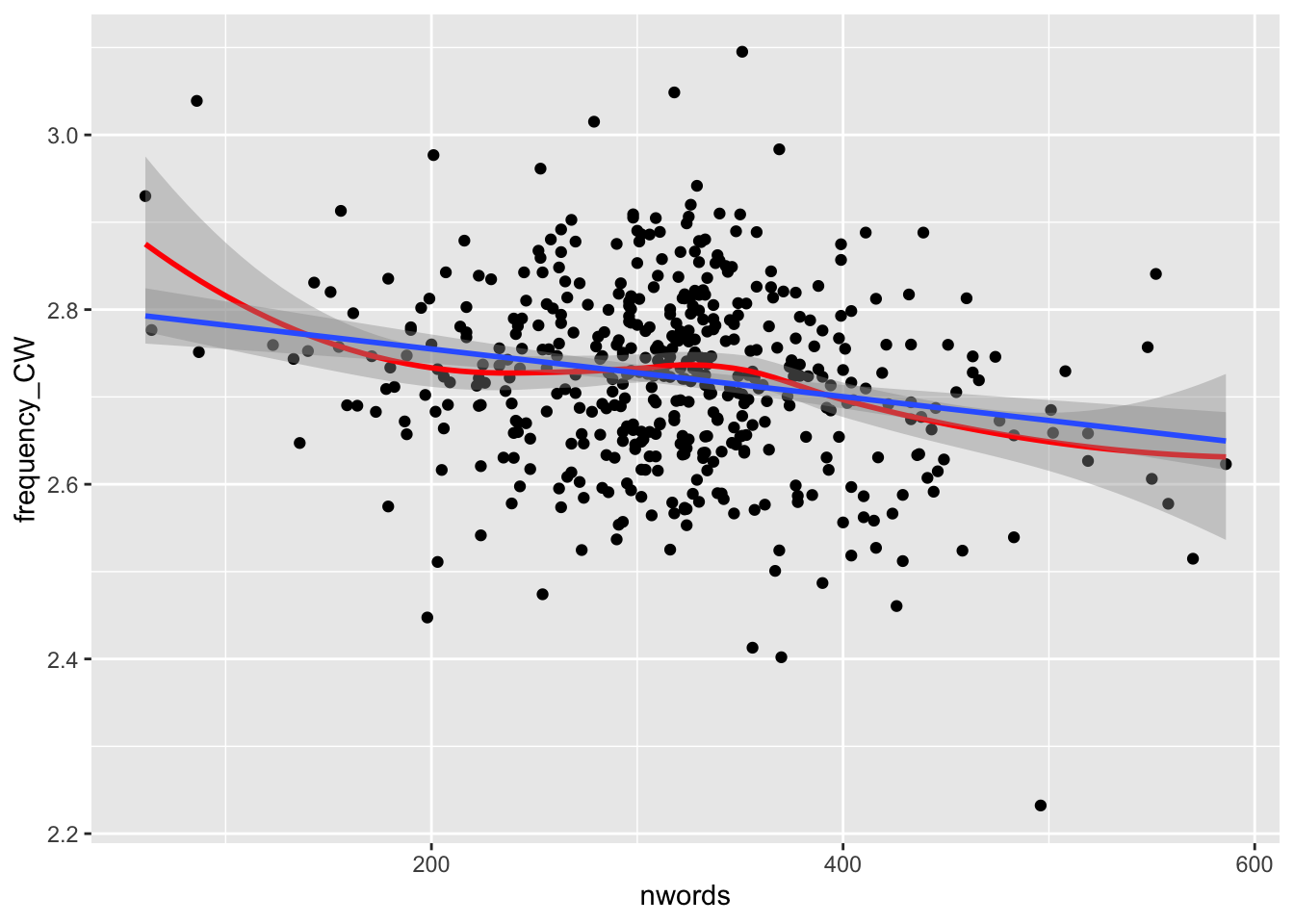## Correlation: Examining the relationship between variables

### The basics

A correlation is a measure that indicates the degree to which the values of one variable change with respect to another variable. For example, we might examine the relationship between the temperature and the number of students wearing a sweatshirt/jacket on campus. In second language learning, we might look at the relationship between the number of hours a student spends studying and their scores on a proficiency test. For most of this tutorial, we will be looking at a common type of correlational analysis (Pearson’s product-moment correlation), though the calculation of other correlation coefficients will be briefly discussed at the end.

Importantly, correlation values (hereafter referred to as r) are an effect size that range from -1.0 to 1.0. When we interpret the size of a correlation, we are concerned with the absolute value of the number, not the directionality. For example, an r value of -.700 is bigger than an r value of .300.

The sign of the r value tells us whether the values from variable A increase as the values of variable B increase (resulting in a positive sign) OR if the variable A values decrease as the variable B values increase (a negative sign). For example, we would expect a NEGATIVE correlation between temperature and number of students wearing jackets on campus (as one value goes down [temperature] the other goes up [number of jacket-wearing individuals] and vice versa). On the other hand, we would expect a POSITIVE relationship between the number of hours spent studying a language and their scores on a language proficiency test (as one value goes up [time spent studying] the other also goes up [proficiency scores] and vice versa).

Finally, p values are also calculated for correlation analyses. These values tell us the probability that observed relationship between the variables would be observed if there were actually no relationship between them.

### Assumptions

Pearson’s correlation has the following assumptions:

• The variables must be continuous (see other tests for ordinal or categorical data)

• The variables must have a linear relationship (i.e., do not have a curvilinear or other relationship)

• There are no outliers (or there are only minimal outliers in large samples)

• The variables must have a bivariate normal distribution (note that this is conceptually related to, but different from the normal distributions that we have been discussing so far)

### Checking assumptions

In our first example, we will examine the relationship between between number of words (as a proxy for proficiency) and lexical sophistication (measured as word frequency) in a corpus of argumentative essays written as part of a standardized test of English proficiency.

These variables (and a few others) are included in the “correlation_sample.csv” file included on our Canvas page.

``````library(ggplot2) #load ggplot2
summary(cor_data) #get descriptive statistics for the dataset``````
``````##      Score       pass.fail  Prompt       nwords       frequency_AW
##  Min.   :1.000   fail:223   p1:240   Min.   : 61.0   Min.   :2.963
##  1st Qu.:3.000   pass:257   p2:240   1st Qu.:273.0   1st Qu.:3.187
##  Median :3.500                       Median :321.0   Median :3.237
##  Mean   :3.427                       Mean   :317.7   Mean   :3.234
##  3rd Qu.:4.000                       3rd Qu.:355.2   3rd Qu.:3.284
##  Max.   :5.000                       Max.   :586.0   Max.   :3.489
##   frequency_CW    frequency_FW   bigram_frequency
##  Min.   :2.232   Min.   :3.598   Min.   :1.240
##  1st Qu.:2.656   1st Qu.:3.827   1st Qu.:1.440
##  Median :2.726   Median :3.903   Median :1.500
##  Mean   :2.723   Mean   :3.902   Mean   :1.500
##  3rd Qu.:2.789   3rd Qu.:3.975   3rd Qu.:1.559
##  Max.   :3.095   Max.   :4.235   Max.   :1.755``````

#### Assumption 1: Continuous or ratio data

Our data for each variable is continuous (it is not, for example, categorical), so we can continue with our analysis.

#### Assumption 2: Linearity

To check the linearity of our data, we will create a scatterplot. For our data to meet the criteria of linearity, it will need to fall in roughly a straight line (and not one that is curvilinear).

The blue line below represents the (straight) line of best fit for the data, while the red line represents a line of best fit based on a moving average. In order to meet the assumption of linearity, we want the red line to approximate the blue line. In this case, we can make a pretty strong argument that we meet the assumption of linearity.

``````ggplot(cor_data, aes(x = nwords, y=frequency_CW )) +
geom_point() +
geom_smooth(method = "loess",color = "red") + #this is a line of best fit based on a moving average
geom_smooth(method = "lm") #this is a line of best fit based on the enture dataset``````#### Assumption 3: Minimal outliers

Outliers can strongly affect our correlation coefficents (particularly in small datasets). Because our dataset is fairly large (480 participants), having a few outliers will not be a large problem. To check for outliers, lets take a look at the scatterplot again.

``````ggplot(cor_data, aes(x = nwords, y=frequency_CW )) +
geom_point() +
geom_smooth(method = "loess",color = "red") + #this is a line of best fit based on a moving average
geom_smooth(method = "lm") #this is a line of best fit based on the enture dataset``````